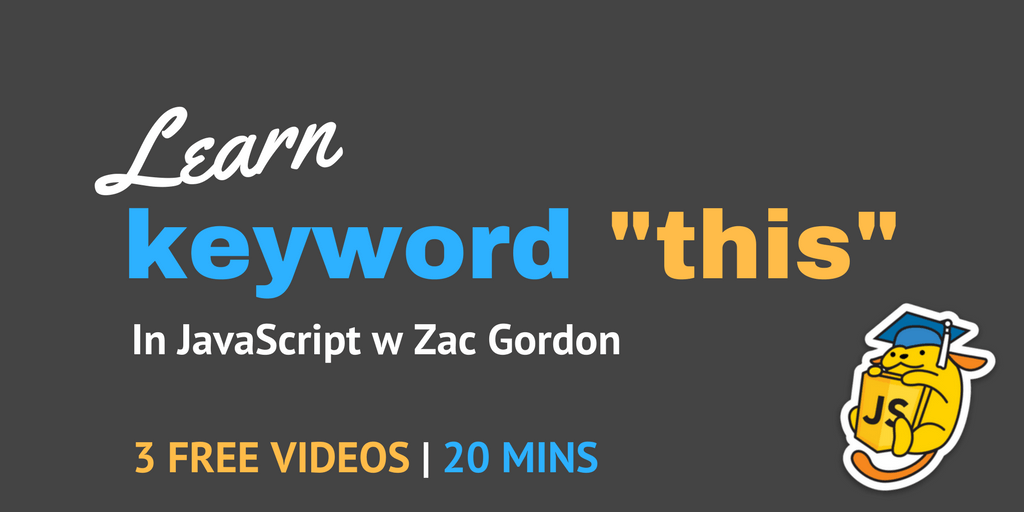One of the important essentials of more advanced JavaScript is how the keyword “this” works in different contexts.

In the three videos below we explore how this works, how you can bind it, and an example of when you might want to bind this in your own code.

## An Introduction to Keyword “this” in JavaScript

In this first lesson we introduce what this is, how it works by default, and when it looses its binding.

You may also want to look into the Mozilla Documentation for this in JavaScript.

## Binding “this” in JavaScript

In this next lesson we look at how you can explicitly bind an object to this with the use of .call(), .apply(), and .bind().

Here again are the Mozilla Docs that also reference .call(), .apply(), and .bind().

## Example of Binding “this” with .addEventListener()

In this final video we give a practical example of when you might want to explicitly bind this to an object.  That is when using Event Listeners.

Here is the example code used in the video above.

## Taking Things Further

In the JavaScript for WordPress Master Course we dive deep into learning JavaScript.  In fact, this introduction to “this” above is just that, an introduction.

If you would like to learn JavaScript more deeply, check out the JavaScript for WordPress Master Course.